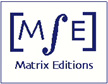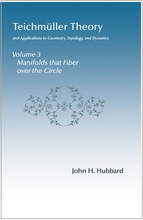Matrix Editions
serious mathematics,
written with the reader in mind

## Sample pages

### Teichmüller Theory Volume 3 Surface Manifolds that fiber over the circleby John H. Hubbard

ISBN 9781943863013
Hardcover, 558 pages

2022, \$95

Index (in pdf)

Sample pages are in pdf. Four pages are provided for each chapter, two for each appendix. These pages are all copyrighted. You are welcome to print one copy for your use.

Chapter 11  Geometry of hyperbolic space
Chapter 12  Rigidity theorems
Chapter 13  Hyperbolization of 3-manifolds that fiber over the circle

Appendix D1  The Nullstellensatz and Selberg's lemma
Appendix D2  The Margulis lemma: another proof
Appendix D3  Fundamental groups, amalgamated sums, and HNN extensions
Appendix D4  Arithmetic Kleinian groups
Appendix D5  Ends of a topological space
Appendix D6  The space of simple geodesics
Appendix D7  Period coordinates
Appendix D8  Ergodic flow, Hopf's argument, and Mostow rigidity
Appendix D9  Teichmüller flow is ergodic
Appendix D10  Minimal but not ergodic
Appendix D11  Sullivan's rigidity theorem
Appendix D12  Fibrations and the Thurston norm